# 2.3 – The Probability Density Function

Previous: 2.2 – A Simple Example

Next: 2.4 – A Simple PDF Example

For many continuous random variables, we can define an extremely useful function with which to calculate probabilities of events associated to the random variable.

Definition: The Probability Density Function
Let F(x) be the distribution function for a continuous random variable X. The probability density function (PDF) for X is given by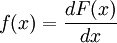wherever the derivative exists.

In short, the PDF of a continuous random variable is the derivative of its CDF. By the Fundamental Theorem of Calculus, we know that the CDF F(x)of a continuous random variable X may be expressed in terms of its PDF: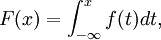where f denotes the PDF of X.

## Properties of the PDF

This formulation of the PDF via the Fundamental Theorem of Calculus allows us to derive the following properties.

Theorem: Properties of the Probability Density Function
If f(x) is a probability density function for a continuous random variable X then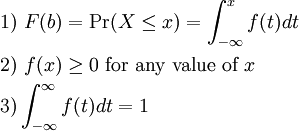The first property, as we have already seen, is just an application of the Fundamental Theorem of Calculus.

The second property states that for a function to be a PDF, it must be nonnegative. This makes intuitive sense since probabilities are always nonnegative numbers. More precisely, we already know that the CDF F(x) is a nondecreasing function of x. Thus, its derivative is f(x) is nonnegative.

The third property states that the area between the function and the x-axis must be 1, or that all probabilities must integrate to 1. This must be true since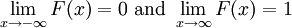; thus 3) follows by the Fundamental Theorem of Calculus.

The PDF gives us a helpful geometrical interpretation of the probability of an event: the probability that a continuous random variable X is less than some value b, is equal to the area under the PDF f(x) on the interval (-∞,b ], as demonstrated in the following graph.

Similarly, we have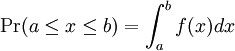.

## What is Pr(X = x)?

Let's now revisit this question that we can interpret probabilities as integrals. It is now clear that for a continuous random variable X, we will always have Pr(X = x) = 0, since the area under a single point of a curve is always zero. In other words, if X is a continuous random variable, the probability that X is equal to a particular value will always be zero. We again note this important difference between continuous and discrete random variables.

## An Important Subtlety

There is an important subtlety in the definition of the PDF of a continuous random variable. Notice that the PDF of a continuous random variable X can only be defined when the distribution function of X is differentiable.

As a first example, consider the experiment of randomly choosing a real number from the interval [0,1]. If we denote this random variable by X, then we see that X is a continuous uniform random variable on [0,1]. Since the likelihood of picking any number is uniform across the interval, we see that the CDF F(x) is given by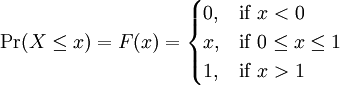This function is differentiable everywhere except at the points x = 0 and x = 1. So the PDF of X is defined at all points except for these two: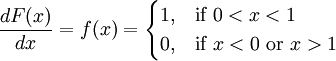Nevertheless, it would still make sense to define the PDF at these points of where the CDF is not differentiable. We know that the integral over a single point is always zero, so we can always change the value of our PDF at any particular point (or at any finite set of points) without changing the probabilities of events associated to our random variable. Thus, we could define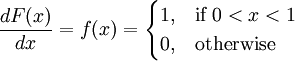or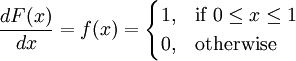Both of these are also PDFs of our continuous uniform random variable X. These two formulations have the advantage of being defined for all real numbers.

## Not All Continuous Random Variables Have PDFs

We can sometimes encounter continuous random variables that simply do not have a meaningful PDF at all. The simplest such example is given by a distribution function called the Cantor staircase.

The Cantor set is defined recursively as follows:

• Delete the middle third of this interval. You are now left with two subintervals [0,1/3) and [2/3,1).
• Delete the middle third of each of these subintervals. Now we have four new subintervals: [0,1/9), [2/9,3/9), [6/9,7/9), and [8/9,1).
• Repeat this middle third deletion for the new subintervals. Continue indefinitely.

If we take this process to the limit, the set that remains is called the Cantor set. It is extremely sparse in [0,1), yet still contains about as many points as the entire interval itself.

We can define a Cantor random variable to have the distribution function that increases on the Cantor set and remains constant off of this set. We define this function as follows:

• Let F(x) be the CDF of our Cantor random variable X. Define F(x) = 0 for x < 0 and F(x) = 1 for x > 1.
• Define F(x) = 1/2 on [1/3,2/3), i.e. on the first middle third deleted in the construction of the Cantor set.
• Define F(x) = 1/4 on [1/9,2/9) and F(x) = 3/4 on [7/9,8/9).
• Define F(x) = 1/8, 3/8, 5/8, and 7/8 on the deleted middle thirds from the third step in our Cantor set construction.
• Continue indefinitely.

After a limiting argument, this procedure defines a continuous function that begins at 0 and increases to 1. However, since this function is constant except on the Cantor set, we see that its derivative off of the Cantor set must be identically zero. On the Cantor set the function is not differentiable and so has no PDF.

What we see is that, for a Cantor random variable, we cannot make any sensible definition for the PDF. It is either identically zero or not defined.

This is an interesting example of how identifying a random variable with its PDF can lead us astray. Thankfully, for the purposes of Math 105, we will never need to consider continuous random variables that do not have PDFs defined everywhere (except possibly at finitely many points).

Previous: 2.2 – A Simple Example

Next: 2.4 – A Simple PDF Example